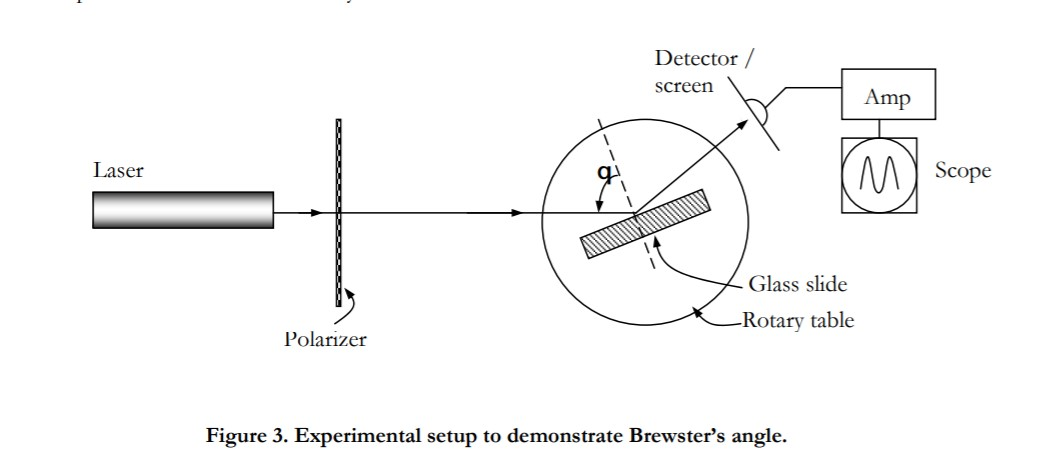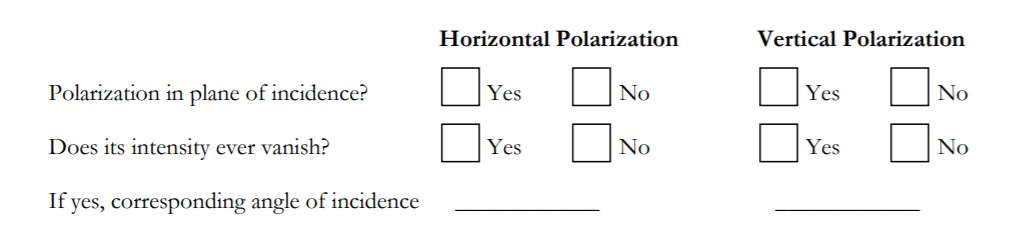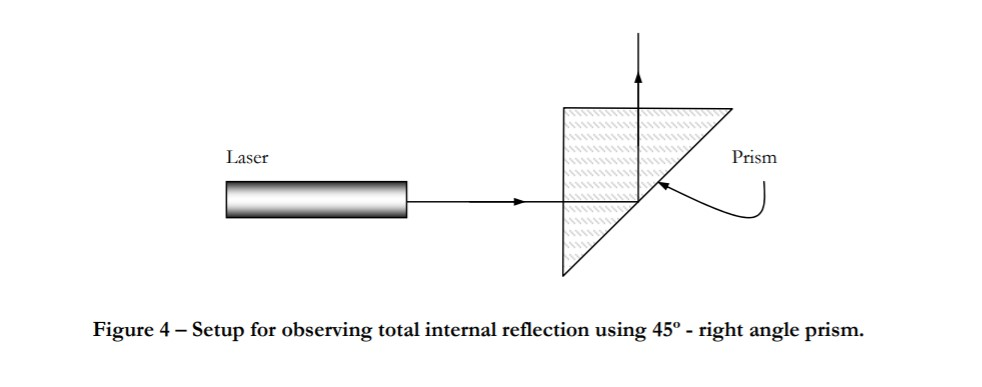# 1.2: In-lab Exercises

•• David Dunmeyer
• Massachusetts Institute of Technology via MIT OpenCourseWare
$$\newcommand{\vecs}{\overset { \rightharpoonup} {\mathbf{#1}} }$$ $$\newcommand{\vecd}{\overset{-\!-\!\rightharpoonup}{\vphantom{a}\smash {#1}}}$$$$\newcommand{\id}{\mathrm{id}}$$ $$\newcommand{\Span}{\mathrm{span}}$$ $$\newcommand{\kernel}{\mathrm{null}\,}$$ $$\newcommand{\range}{\mathrm{range}\,}$$ $$\newcommand{\RealPart}{\mathrm{Re}}$$ $$\newcommand{\ImaginaryPart}{\mathrm{Im}}$$ $$\newcommand{\Argument}{\mathrm{Arg}}$$ $$\newcommand{\norm}{\| #1 \|}$$ $$\newcommand{\inner}{\langle #1, #2 \rangle}$$ $$\newcommand{\Span}{\mathrm{span}}$$ $$\newcommand{\id}{\mathrm{id}}$$ $$\newcommand{\Span}{\mathrm{span}}$$ $$\newcommand{\kernel}{\mathrm{null}\,}$$ $$\newcommand{\range}{\mathrm{range}\,}$$ $$\newcommand{\RealPart}{\mathrm{Re}}$$ $$\newcommand{\ImaginaryPart}{\mathrm{Im}}$$ $$\newcommand{\Argument}{\mathrm{Arg}}$$ $$\newcommand{\norm}{\| #1 \|}$$ $$\newcommand{\inner}{\langle #1, #2 \rangle}$$ $$\newcommand{\Span}{\mathrm{span}}$$

## Safety, Polarization States of Optical Waves, Dielectric Reflection and Geometric Optics

### 1.1 Linear Polarizer

1. As was discussed in lecture, light reflected off the waxed floor tiles is predominantly polarized perpendicular to the plane of incidence. Holding the given sheet of linear polarizer close to your eye, use such reflections to determine the axis of the polarizer. Convince the T.A. that you have identified the polarizer axis.
2. Explain using electric dipole arguments and with the aid of diagrams why the light reflected from the floor wax is predominantly polarized perpendicular to the plane of incidence.

### 1.2 Two-Polarizer Diagnostic System

The setup you are given consists of a randomly polarized (unpolarized) laser beam of intensity I0, a pair of polarizers, and a screen arranged as illustrated in Figure 2. The second polarizer is often called an analyzer. Its axis is generally oriented perpendicular (crossed) or parallel to the axis of the first polarizer.1. With the first and second polarizers crossed in the above system, place a third sheet of linear polarizer in the system between the two stationary crossed sheets and observe the intensity of the transmitted light on the screen as you rotate the third polarizer. Make sure to record the orientation of every element in the diagnostic system in your lab notebook. Now place a photodetector at D and record below the intensity on the screen as a function of the angle of rotation of the third polarizer. About 10 points between (and including) two adjacent intensity maxima should be sufficient. Record this chart into your lab notebook.
 Angle Relative Intensity
2. Explain why the intensity on the screen changes as the central polarizer is rotated?
3. Derive the form of the theoretically expected intensity at the detector, and compare it with your measurement (you may use Jones matrices for this, or use basic trigonometry). Explain the discrepancy, if any.

### 1.3 Quarter and Half Wave Plates

1. You are given one quarter-wave plate and one half-wave plate (labeled A and B) but it is not known which plate is which. Use the system in Fig. 1 to: (i) find the axes of the quarter-wave plate, (ii) find the axes of the half-wave plate, and (iii) distinguish one plate from the other. Convince the T.A. that you have indeed found the axes and distinguished the two wave plates. Describe the experiments you performed on the two wave plates. Tabulate the results, and explain how you arrived at your conclusions. Make sure to record the orientation of every element in the diagnostic system in your lab notebooks.
2. A half-wave plate can be used to convert vertically polarized light into horizontally polarized light, and vice versa. Another useful application of a half-wave plate is as a linear polarization rotator. Indeed, if linear polarized light is incident at normal incidence on a half-wave plate and the plate is rotated through an angle q about an axis parallel with the propagation direction, the polarization of the transmitted beam is rotate by 2q. In your lab notebook, draw diagrams and explain how (1): a half-wave plate converts vertically polarized light to horizontally polarized light, and (2) how and why a half-wave plate achieves polarization rotation. (We are not looking for detailed calculations, but instead a detailed written description using diagrams as visual aids.)

### 1.4 Magic Sheet

You are given a sheet of "magic" material, which transmits light when folded in one direction, but blocks light when folded in the other direction. Observe this for yourself. Using only linear polarizers, devise nondestructive experiments to determine the possible optical elements that constitute the "magic" material. Record your data in tabular form in your lab notebook, and explain with the help of diagrams why light is blocked or passed in each experiment you perform. Show clearly how you arrive at your conclusion about the magic material.

### 1.5 Dielectric Reflection and the Brewster Angle

The Brewster angle is defined as the angle of incidence for which the reflected light intensity from a dielectric boundary goes to zero for TM polarization. This effect can be demonstrated by the experiment shown schematically below.Use a randomly polarized laser beam for this experiment. Align the glass slide so that its axis of rotation is perpendicular to the laser beam. This can be accomplished by adjusting the laser or the glass slide so that at normal incidence, the reflected beam goes back close to or into the laser cavity.

1. Where is the plane of incidence in your experiment?
2. With the linear polarizer aligned with its axis horizontal, and the laser beam centered on the axis of rotation of the glass slide, rotate the glass slide and observe the intensity of the reflected beam on the screen. Rotate the polarizer sheet by 90± and repeat the experiment. Copy the table below into your lab notebook. Record your observations of the reflected beam for each of the two polarization cases in your lab notebook.3. Compute the index of refraction of the glass slide from your measurement of the angle. The wavelength of the He-Ne laser is 633 nm. Comment on the accuracy of this method of measuring refractive index.
4. Now, use the photodetector to measure the reflectivity of the glass slide as a function of the angle of incidence for both polarizations. About every 5º is good enough (the most accurate measurements are obtained if measurements for both polarizations are made before the angle of incidence is changed). Record the raw data in your lab notebook in a similar fashion to the table shown below. Plot your data using a software package of your choice, and paste it into your lab notebook.

TM Polarization

 Angle of Incidence Relative Intensity

TE Polarization

 Angle of Incidence Relative Intensity

### 1.6 Total Internal Reflection System

You are given a 45º-right-angle glass prism on a rotary table and a HeNe laser set up in the arrangement shown in the Figure 4. Illuminate one of the small faces with the laser beam at normal incidence (reflection off this surface goes back into the laser cavity) and observe the phenomenon of total internal reflection. Slowly rotate the prism until the transmitted beam just grazes the hypotenuse of the prism. Measure the angle of rotation, and use it to calculate the refractive index of the glass block. You should have come up with an equation to do this in the pre-lab exercises. Use your derived equation, while in the Lab to perform a reality check on the index of refraction.### 1.7 (Part 1) Imaging Properties of a Convex Lens

In this exercise, we will explore the imaging properties of a simple symmetric biconvex lens. The setup we will use is shown in Figure 1. It consists of a white light source a transmission object (slide), a biconvex lens, and a screen, all mounted along an optical rail.Using the setup in Fig. 1, and keeping the light source (slide) and object (lamp) fixed, select five different object distances, O, (distance from the lens to the object) by moving the lens. For each object distance, measure: (1) the corresponding image distance, I, for sharply defined image on the screen, and (2) the size of the image.

1. Make a plot of 1/I versus 1/O and from your plot determine the focal length of the lens (use all your data points – if you are not sure how to fit the data, please ask). Include the raw data, the plot and your calculation of the focal length in your report.
2. If the refractive index of the lens is 1.52, what is the radius of curvature of its convex surfaces?
3. Why is a sharp image not seen when the object distance is less than the focal length of the lens?
4. If two lenses of focal length F1 and F2 are abutted, what is the focal length of the combination? Show your calculation.
5. How would you go about measuring the focal length of a biconcave lens?

### 1.7 (Part 2) Optional for Extra Credit

Build the telescope you designed in the pre-lab exercise. Comment on the performance of your telescope. Did your telescope operate as you expected? Why or why not? Explain the operation of your telescope to the TA.

Congratulations, you finished the in-lab portion of Laboratory #1. Please write down in your notebook how long it took (start to finish) to complete: (a) the Pre-lab, (b) the in-lab portion, and (c) the postlab writeup.

This page titled 1.2: In-lab Exercises is shared under a CC BY-NC-SA 4.0 license and was authored, remixed, and/or curated by David Dunmeyer (MIT OpenCourseWare) .